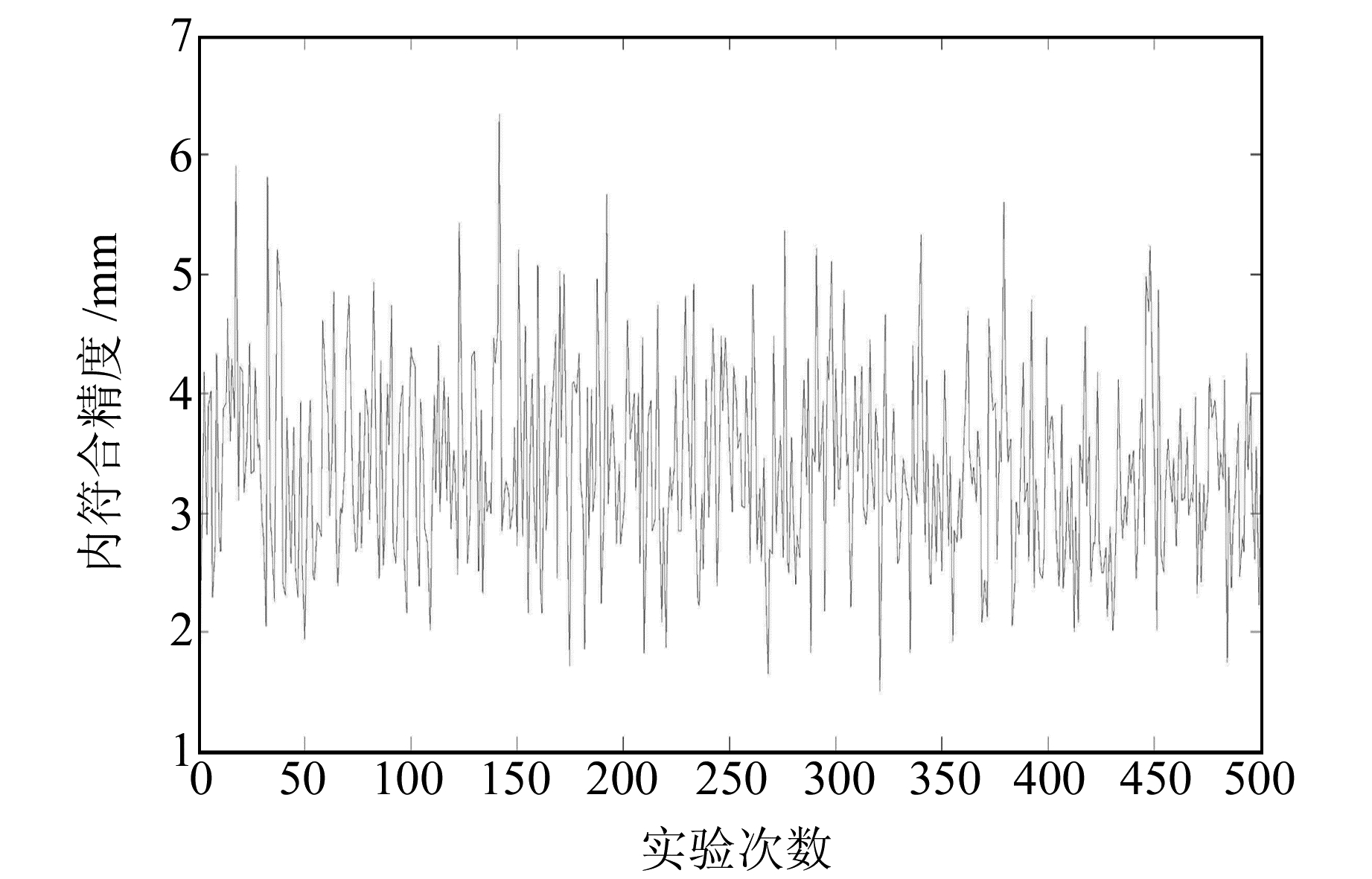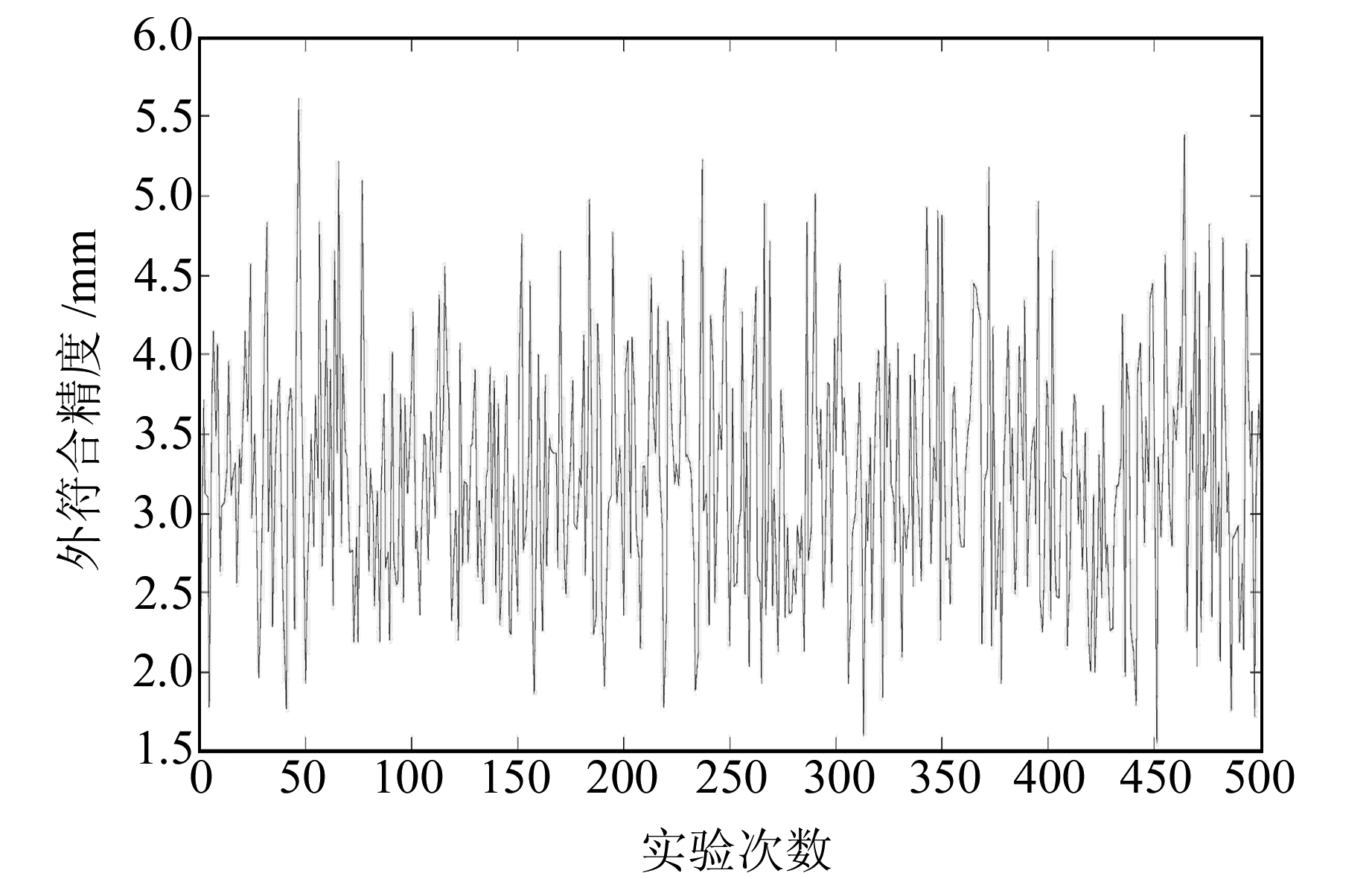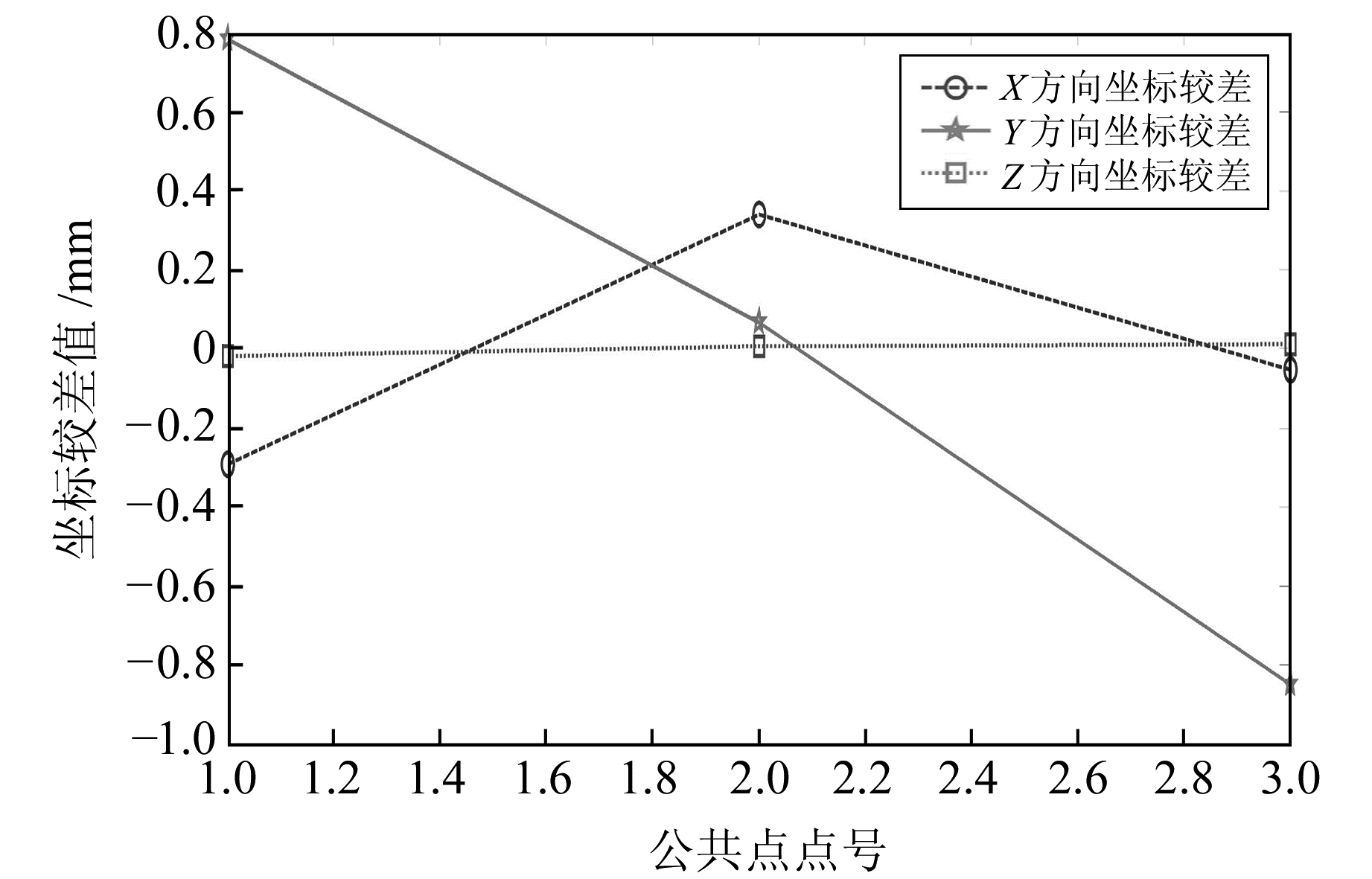﻿ 基于实四元数的大旋转角三维坐标转换的改进谱修正迭代解法文章快速检索 高级检索
 大地测量与地球动力学2019, Vol. 39Issue (11): 1182-1187  DOI: 10.14075/j.jgg.2019.11.016### 引用本文JIANG Pan, YOU Wei. The Improved Iteration Method by Correcting Characteristic Value for Transformation of Three-Dimensional Coordinates Based on Large Rotation Angle and Quaternions[J]. Journal of Geodesy and Geodynamics, 2019, 39(11): 1182-1187.### Foundation support

National Natural Science Foundation of China, No. 41574018, 41604068, 41404018.

### Corresponding author

YOU Wei, PhD, associate professor, majors in satellite gravimetry and measurement data processing, E-mail:youwei1985@foxmail.com.

### 第一作者简介

JIANG Pan, postgraduate, majors in geodetic and measurement data processing, E-mail: macfreestream@foxmail.com.

### 文章历史

1. 西南交通大学地球科学与环境工程学院，成都市犀安路999号，611756;
2. 高速铁路运营安全空间信息技术国家地方联合工程实验室，成都市二环路北一段111号，610031

1 单位实四元数与三维坐标转换

 $\left[ {\begin{array}{*{20}{c}} {{{X'}_i}}\\ {{{Y'}_i}}\\ {{{Z'}_i}} \end{array}} \right] = \left[ {\begin{array}{*{20}{c}} {{X_0}}\\ {{Y_0}}\\ {{Z_0}} \end{array}} \right] + (1 + \mu )\mathit{\boldsymbol{R}}\left[ {\begin{array}{*{20}{c}} {{X_i}}\\ {{Y_i}}\\ {{Z_i}} \end{array}} \right]$ (1)

 $\begin{array}{c} \mathit{\boldsymbol{R}} = {\mathit{\boldsymbol{R}}_X}(\alpha ){\mathit{\boldsymbol{R}}_Y}(\beta ){\mathit{\boldsymbol{R}}_Z}(\gamma ) = \\ \left[ {\begin{array}{*{20}{c}} 1&0&0\\ 0&{\cos \alpha }&{\sin \alpha }\\ 0&{ - \sin \alpha }&{\cos \alpha } \end{array}} \right]\left[ {\begin{array}{*{20}{c}} {\cos \beta }&0&{ - \sin \beta }\\ 0&1&0\\ {\sin \beta }&0&{\cos \beta } \end{array}} \right]\\ \left[ {\begin{array}{*{20}{c}} {\cos \gamma }&{\sin \gamma }&0\\ { - \sin \gamma }&{\cos \gamma }&0\\ 0&0&1 \end{array}} \right] \end{array}$ (2)

 $\mathit{\boldsymbol{R}} = \left[ {\begin{array}{*{20}{c}} {q_0^2 + q_1^2 - q_2^2 - q_3^2}&{2({q_1}{q_2} - {q_0}{q_3})}&{2({q_1}{q_3} + {q_0}{q_2})}\\ {2({q_1}{q_2} + {q_0}{q_3})}&{q_0^2 - q_1^2 + q_2^2 - q_3^2}&{2({q_2}{q_3} - {q_0}{q_1})}\\ {2({q_1}{q_3} - {q_0}{q_2})}&{2({q_2}{q_3} + {q_0}{q_1})}&{q_0^2 - q_1^2 - q_2^2 + q_3^2} \end{array}} \right]$ (3)

 $q_{0}^{2}+q_{1}^{2}+q_{2}^{2}+q_{3}^{2}=1$ (4)

 ${\mathit{\boldsymbol{V}}_i} = {\mathit{\boldsymbol{A}}_i}\mathit{\boldsymbol{\hat x}} - {\mathit{\boldsymbol{L}}_i}, i = 1, 2, \cdots , n$ (5)

 $\mathit{\boldsymbol{B\hat x}} + \mathit{\boldsymbol{W}} = 0$ (6)

N=ATPA，权阵P一般设为单位阵，利用拉格朗日乘数法按附有限制条件的间接平差解算式(5)和式(6)，得到法方程：

 $\left[ {\begin{array}{*{20}{c}} \mathit{\boldsymbol{N}}&{{\mathit{\boldsymbol{B}}^T}}\\ \mathit{\boldsymbol{B}}&0 \end{array}} \right]\left[ {\begin{array}{*{20}{c}} {\mathit{\boldsymbol{\hat x}}}\\ \mathit{\boldsymbol{K}} \end{array}} \right] = \left[ {\begin{array}{*{20}{c}} {{\mathit{\boldsymbol{A}}^T}\mathit{\boldsymbol{PL}}}\\ { - \mathit{\boldsymbol{W}}} \end{array}} \right]$ (7)

2 病态问题的岭估计求解

 $\mathit{\boldsymbol{L}} = \mathit{\boldsymbol{AX}} + \mathit{\boldsymbol{ \boldsymbol{\varDelta} }}$ (8)

 ${\mathit{\boldsymbol{\hat x}}_{LS}} = {({\mathit{\boldsymbol{A}}^T}\mathit{\boldsymbol{PA}})^{ - 1}}({\mathit{\boldsymbol{A}}^T}\mathit{\boldsymbol{PL}})$ (9)

 $\mathit{\boldsymbol{\hat x}} = {({\mathit{\boldsymbol{A}}^T}\mathit{\boldsymbol{PA}} + \alpha \mathit{\boldsymbol{I}})^{ - 1}}({\mathit{\boldsymbol{A}}^T}\mathit{\boldsymbol{PL}})$ (10)

3 基于岭参数的谱修正迭代法 3.1 谱修正迭代法

 ${\mathit{\boldsymbol{\hat X}}^{(n)}} = {({\mathit{\boldsymbol{A}}^T}\mathit{\boldsymbol{PA}} + \mathit{\boldsymbol{I}})^{ - 1}}({\mathit{\boldsymbol{A}}^T}\mathit{\boldsymbol{PL}} + {\mathit{\boldsymbol{\hat X}}^{(n - 1)}})$ (11)

3.2 改进谱修正迭代法

 ${\mathit{\boldsymbol{\hat X}}^{(n)}} = {({\mathit{\boldsymbol{A}}^T}\mathit{\boldsymbol{PA}} + (\alpha + 1)\mathit{\boldsymbol{I}})^{ - 1}}({\mathit{\boldsymbol{A}}^T}\mathit{\boldsymbol{PL}} + {\mathit{\boldsymbol{\hat X}}^{(n - 1)}})$ (12)

 ${\mathit{\boldsymbol{\hat X}}^{(n)}} = {({\mathit{\boldsymbol{A}}^T}\mathit{\boldsymbol{PA}} + \mathit{\boldsymbol{K}})^{ - 1}}({\mathit{\boldsymbol{A}}^T}\mathit{\boldsymbol{PL}} + \mathit{\boldsymbol{K}}{\mathit{\boldsymbol{\hat X}}^{(n - 1)}})$ (13)

 ${\mathit{\boldsymbol{\hat X}}^{(n)}} = {({\mathit{\boldsymbol{A}}^T}\mathit{\boldsymbol{PA}} + \alpha \mathit{\boldsymbol{T}})^{ - 1}}({\mathit{\boldsymbol{A}}^T}\mathit{\boldsymbol{PL}} + \alpha \mathit{\boldsymbol{T}}{\mathit{\boldsymbol{\hat X}}^{(n - 1)}})$ (14)

3.3 三维坐标转换模型求解

 $\left[ {\begin{array}{*{20}{c}} \mathit{\boldsymbol{N}}&{{\mathit{\boldsymbol{B}}^T}}\\ \mathit{\boldsymbol{B}}&0 \end{array}} \right] = \mathit{\boldsymbol{M}}, \left[ {\begin{array}{*{20}{c}} {\mathit{\boldsymbol{\hat x}}}\\ \mathit{\boldsymbol{K}} \end{array}} \right] = \mathit{\boldsymbol{Y}}, \left[ {\begin{array}{*{20}{c}} {{\mathit{\boldsymbol{A}}^T}\mathit{\boldsymbol{PL}}}\\ { - \mathit{\boldsymbol{W}}} \end{array}} \right] = \mathit{\boldsymbol{U}}$ (15)

 $\mathit{\boldsymbol{MY}} = \mathit{\boldsymbol{U}}$ (16)

 $(\boldsymbol{M}+\alpha \boldsymbol{T}) \boldsymbol{Y}=\boldsymbol{U}+\alpha \boldsymbol{T} \boldsymbol{Y}$ (17)

 $\hat{\boldsymbol{Y}}^{(k)}=(\boldsymbol{M}+\alpha \boldsymbol{T})^{-1}\left(\boldsymbol{U}+\alpha \boldsymbol{T} \hat{\boldsymbol{Y}}^{(k-1)}\right)$ (18)

4 算例与分析 4.1 算例1表 1 公共点坐标数据 Tab. 1 Common point coordinate data表 2 模型转换参数(真值) Tab. 2 Model conversion parameters(true value)

1) 对未知参数的初始值采用以下3种不同的取值方案。

2) 采用本文提出的算法和文献算法(算法二)，使表 1中的公共点全部参与解算，得到的结果见表 3表 3 转换参数的计算结果 Tab. 3 The results of transformation parameter after iterative calculation

3) 初值取为1)中3种方案时，本文算法和文献算法(算法二)的机器耗时分别如表 4(单位s)所示，结果均利用MATLAB编程计算得到。表 4 不同方案两种算法机器耗时 Tab. 4 Two algorithm machines take time in different scenarios

4.2 算例2图 1 内符合精度 Fig. 1 Inner precision图 2 外符合精度 Fig. 2 External precision

4.3 算例3图 3 坐标较差 Fig. 3 Coordinate difference

5 结语

1) 本文将三维旋转变换矩阵用四元数进行表示，构造了基于单位实四元数的三维坐标变换模型，并引入岭参数和泛函矩阵，得到改进的谱修正迭代算法。相对于用方向余弦、欧拉角和罗德里格矩阵构造的三维旋转变换矩阵，实四元数几何意义更加直观，且采用单位实四元数构造旋转矩阵无需进行复杂的三角函数求导，易于线性化，系数矩阵更为简洁。

2) 在进行三维坐标转换时，由于选取的公共点都是非均匀分布且都在局部区域，转换模型法方程矩阵的条件数较大，即法方程矩阵存在严重的病态性。考虑到模型法方程矩阵的病态性，引入岭参数和泛函矩阵改进谱修正迭代法求解转换参数，可有效降低法方程病态问题带来的不利影响，使方程求解达到稳定，同时方程迭代求解时解的估计值接近真值的程度较谱修正迭代法高。

3) 通过模拟数据和实测数据对算法进行验证，并与其他算法进行比较表明，本文算法具有收敛速度快、不依赖转换参数初值、全局收敛、解是无偏的、便于程序实现等特点。

  潘国荣, 汪大超, 周跃寅. 两种大转角空间坐标转换模型研究[J]. 山东科技大学学报:自然科学版, 2015, 34(1): 61-67 (Pan Guorong, Wang Dachao, Zhou Yueyin. Two Spatial Coordinate Transformation Model of Large Angle[J]. Journal of Shandong University of Science and Technology:Natural Science, 2015, 34(1): 61-67) (0)  姚宜斌, 黄承猛, 李程春, 等. 一种适用于大角度的三维坐标转换参数求解算法[J]. 武汉大学学报:信息科学版, 2012, 37(3): 253-256 (Yao Yibin, Huang Chengmeng, Li Chengchun, et al. A New Algorithm for Solution of Transformation Parameters of Big Rotation Angle's 3D Coordinate[J]. Geomatics and Information Science of Wuhan University, 2012, 37(3): 253-256) (0)  陈义, 沈云中, 刘大杰. 适用于大旋转角的三维基准转换的一种简便模型[J]. 武汉大学学报:信息科学版, 2004, 29(12): 1101-1104 (Chen Yi, Shen Yunzhong, Liu Dajie. A Simplified Model of Three Dimensional-Datum Transformation Adapted to Big Rotation Angle[J]. Geomatics and Information Science of Wuhan University, 2004, 29(12): 1101-1104) (0)  吕志鹏, 伍吉仓, 公羽. 利用四元数改进大旋转角坐标变换模型[J]. 武汉大学学报:信息科学版, 2016, 41(4): 547-553 (Lü Zhipeng, Wu Jicang, Gong Yu. Improvement of a Three-Dimensional Coordinate Transformation Model Adapted to Big Rotation Angle Based on Quaternion[J]. Geomatics and Information Science of Wuhan University, 2016, 41(4): 547-553) (0)  姚吉利, 韩保民, 杨元喜. 罗德里格矩阵在三维坐标转换严密解算中的应用[J]. 武汉大学学报:信息科学版, 2006, 31(12): 1094-1096 (Yao Jili, Han Baomin, Yang Yuanxi. Applications of Lodrigues Matrix in 3D Coordinate Transformation[J]. Geomatics and Information Science of Wuhan University, 2006, 31(12): 1094-1096) (0)  王新洲. 非线性模型参数估计理论与应用[M]. 武汉: 武汉大学出版社, 2002 (Wang Xinzhou. Theory and Application of Parameter Estimation of Nonlinear Model[M]. Wuhan: Wuhan University Press, 2002) (0)  Hoerl A E, Kennard R W. Ridge Regression: Biased Estimation for Non-Orthogonal Problem[J]. Technometrics, 1970, 12(1): 55-67 DOI:10.1080/00401706.1970.10488634 (0)  王新洲, 刘丁酉, 黄海兰. 谱修正迭代结果的协因素矩阵[J]. 武汉大学学报:信息科学版, 2003, 28(4): 429-431 (Wang Xinzhou, Liu Dingyou, Huang Hailan. The Co-Factor Matrix of the Iteration Method by Correcting Characteristic Value[J]. Geomatics and Information Science of Wuhan University, 2003, 28(4): 429-431) (0)  王新洲, 刘丁酉, 张前勇, 等. 谱修正迭代法及其在测量数据处理中的应用[J]. 黑龙江工程学院学报, 2001, 15(2): 3-6 (Wang Xinzhou, Liu Dingyou, Zhang Qianyong, et al. The Iteration by Correcting Characteristic Value and Its Application in Surveying Data Processing[J]. Journal of Heilongjiang Institute of Technology, 2001, 15(2): 3-6 DOI:10.3969/j.issn.1671-4679.2001.02.001) (0)  Hamilton S W R. On Quaternions; or on a New System of Imaginaries in Algebra[J]. Philosophical Magazine, 2009, 29(191): 425-439 (0)  李亚萍, 黄崇超. 实四元数与三维旋转[J]. 武汉水利电力大学学报, 1995, 28(6): 607-612 (Li Yaping, Huang Chongchao. Using Real Quaternions to Represent Rotation in Three Dimensions[J]. Journal of Wuhan University of Hydraulic and Electric Engineering, 1995, 28(6): 607-612) (0)  李德仁, 袁修孝. 误差处理与可靠性理论[M]. 武汉: 武汉大学出版社, 2002 (Li Deren, Yuan Xiuxiao. Error Processing and Reliability Theory[M]. Wuhan: Wuhan University Press, 2002) (0)  王振杰.大地测量中不适定问题的正则化解法研究[D].武汉: 中国科学院测量与地球物理研究所, 2002 (Wang Zhenjie. Research on the Regularization Solutions of Ill-Posed Problems in Geodesy[D].Wuhan: Institute of Geodesy and Geophysics, CAS, 2002) http://cdmd.cnki.com.cn/article/cdmd-80057-2004041260.htm (0)  徐文, 陈义. 迭代法在病态问题中的应用[J]. 工程勘察, 2016(8): 71-73 (Xu Wen, Chen Yi. Application of Iterative Method in Ill-Posed Problems[J]. Geotechnical Investigation and Surveying, 2016(8): 71-73) (0)  刘斌, 龚健雅, 江万寿, 等. 基于岭参数的谱修正迭代法及其在有理多项式参数求解中的应用[J]. 武汉大学学报:信息科学版, 2012, 37(4): 399-402 (Liu Bin, Gong Jianya, Jiang Wanshou, et al. Improvement of the Iteration by Correcting Characteristic Value Based on Ridge Estimation and Its Application in RPC Calculating[J]. Geomatics and Information Science of Wuhan University, 2012, 37(4): 399-402) (0)  王振杰, 欧吉坤. 用L-曲线法确定岭估计中的岭参数[J]. 武汉大学学报:信息科学版, 2004, 29(3): 235-238 (Wang Zhenjie, Ou Jikun. Determining the Ridge Parameter in a Ridge Estimation Using L-Curve Method[J]. Geomatics and Information Science of Wuhan University, 2004, 29(3): 235-238) (0)
The Improved Iteration Method by Correcting Characteristic Value for Transformation of Three-Dimensional Coordinates Based on Large Rotation Angle and Quaternions
JIANG Pan1YOU Wei1,21. Faculty of Geosciences and Environmental Engineering, Southwest Jiaotong University, 999 Xi'an Road, Chengdu 611756, China;
2. State-Province Joint Engineering Laboratory of Spatial Information Technology for High-Speed Railway Safety, 111 First Section of North-Erhuan Road, Chengdu 610031, China
Abstract: We propose a new method for ill-posed problems in the large rotation angle three-dimensional coordinate transformation based on the unit quaternion. This method constructs a rotation matrix by unit quaternion, which avoids complex trigonometric function derivations, is easy to linearize, and has a more concise coefficient matrix. Considering the ill-posedness of the normal equation matrix, the ridge parameter and the functional matrix are introduced, which reduces the adverse effects caused by the ill-posedness of the equation, and generates a stable equation solution. At the same time, the estimated value of the solution when the equation is iteratively solved is closer to the true value than the traditional iteration method by correcting characteristic value. The algorithm is verified by simulation and measured data, showing that the algorithm has the characteristics of fast convergence, no initial value of conversion parameters, global convergence, unbiased solution and easy program implementation. It provides a new way for general coordinate transformation.
Key words: quaternion; ill-posed problem; the iteration method by correcting characteristic value; ridge parameter